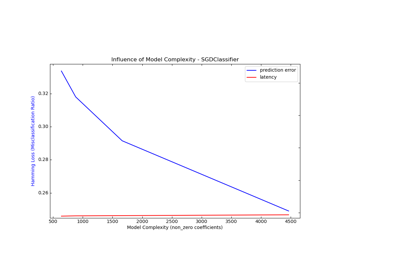# sklearn.svm.NuSVR¶

class sklearn.svm.NuSVR(*, nu=0.5, C=1.0, kernel='rbf', degree=3, gamma='scale', coef0=0.0, shrinking=True, tol=0.001, cache_size=200, verbose=False, max_iter=-1)[source]

Nu Support Vector Regression.

Similar to NuSVC, for regression, uses a parameter nu to control the number of support vectors. However, unlike NuSVC, where nu replaces C, here nu replaces the parameter epsilon of epsilon-SVR.

The implementation is based on libsvm.

Read more in the User Guide.

Parameters
nufloat, default=0.5

An upper bound on the fraction of training errors and a lower bound of the fraction of support vectors. Should be in the interval (0, 1]. By default 0.5 will be taken.

Cfloat, default=1.0

Penalty parameter C of the error term.

kernel{‘linear’, ‘poly’, ‘rbf’, ‘sigmoid’, ‘precomputed’}, default=’rbf’

Specifies the kernel type to be used in the algorithm. It must be one of ‘linear’, ‘poly’, ‘rbf’, ‘sigmoid’, ‘precomputed’ or a callable. If none is given, ‘rbf’ will be used. If a callable is given it is used to precompute the kernel matrix.

degreeint, default=3

Degree of the polynomial kernel function (‘poly’). Ignored by all other kernels.

gamma{‘scale’, ‘auto’} or float, default=’scale’

Kernel coefficient for ‘rbf’, ‘poly’ and ‘sigmoid’.

• if gamma='scale' (default) is passed then it uses 1 / (n_features * X.var()) as value of gamma,

• if ‘auto’, uses 1 / n_features.

Changed in version 0.22: The default value of gamma changed from ‘auto’ to ‘scale’.

coef0float, default=0.0

Independent term in kernel function. It is only significant in ‘poly’ and ‘sigmoid’.

shrinkingbool, default=True

Whether to use the shrinking heuristic. See the User Guide.

tolfloat, default=1e-3

Tolerance for stopping criterion.

cache_sizefloat, default=200

Specify the size of the kernel cache (in MB).

verbosebool, default=False

Enable verbose output. Note that this setting takes advantage of a per-process runtime setting in libsvm that, if enabled, may not work properly in a multithreaded context.

max_iterint, default=-1

Hard limit on iterations within solver, or -1 for no limit.

Attributes
support_ndarray of shape (n_SV,)

Indices of support vectors.

support_vectors_ndarray of shape (n_SV, n_features)

Support vectors.

dual_coef_ndarray of shape (1, n_SV)

Coefficients of the support vector in the decision function.

coef_ndarray of shape (1, n_features)

Weights assigned to the features (coefficients in the primal problem). This is only available in the case of a linear kernel.

coef_ is readonly property derived from dual_coef_ and support_vectors_.

intercept_ndarray of shape (1,)

Constants in decision function.

NuSVC

Support Vector Machine for classification implemented with libsvm with a parameter to control the number of support vectors.

SVR

epsilon Support Vector Machine for regression implemented with libsvm.

Notes

Examples

>>> from sklearn.svm import NuSVR
>>> from sklearn.pipeline import make_pipeline
>>> from sklearn.preprocessing import StandardScaler
>>> import numpy as np
>>> n_samples, n_features = 10, 5
>>> np.random.seed(0)
>>> y = np.random.randn(n_samples)
>>> X = np.random.randn(n_samples, n_features)
>>> regr = make_pipeline(StandardScaler(), NuSVR(C=1.0, nu=0.1))
>>> regr.fit(X, y)
Pipeline(steps=[('standardscaler', StandardScaler()),
('nusvr', NuSVR(nu=0.1))])


Methods

 fit(X, y[, sample_weight]) Fit the SVM model according to the given training data. get_params([deep]) Get parameters for this estimator. Perform regression on samples in X. score(X, y[, sample_weight]) Return the coefficient of determination R^2 of the prediction. set_params(**params) Set the parameters of this estimator.
__init__(*, nu=0.5, C=1.0, kernel='rbf', degree=3, gamma='scale', coef0=0.0, shrinking=True, tol=0.001, cache_size=200, verbose=False, max_iter=-1)[source]

Initialize self. See help(type(self)) for accurate signature.

fit(X, y, sample_weight=None)[source]

Fit the SVM model according to the given training data.

Parameters
X{array-like, sparse matrix} of shape (n_samples, n_features) or (n_samples, n_samples)

Training vectors, where n_samples is the number of samples and n_features is the number of features. For kernel=”precomputed”, the expected shape of X is (n_samples, n_samples).

yarray-like of shape (n_samples,)

Target values (class labels in classification, real numbers in regression)

sample_weightarray-like of shape (n_samples,), default=None

Per-sample weights. Rescale C per sample. Higher weights force the classifier to put more emphasis on these points.

Returns
selfobject

Notes

If X and y are not C-ordered and contiguous arrays of np.float64 and X is not a scipy.sparse.csr_matrix, X and/or y may be copied.

If X is a dense array, then the other methods will not support sparse matrices as input.

get_params(deep=True)[source]

Get parameters for this estimator.

Parameters
deepbool, default=True

If True, will return the parameters for this estimator and contained subobjects that are estimators.

Returns
paramsmapping of string to any

Parameter names mapped to their values.

predict(X)[source]

Perform regression on samples in X.

For an one-class model, +1 (inlier) or -1 (outlier) is returned.

Parameters
X{array-like, sparse matrix} of shape (n_samples, n_features)

For kernel=”precomputed”, the expected shape of X is (n_samples_test, n_samples_train).

Returns
y_predndarray of shape (n_samples,)
score(X, y, sample_weight=None)[source]

Return the coefficient of determination R^2 of the prediction.

The coefficient R^2 is defined as (1 - u/v), where u is the residual sum of squares ((y_true - y_pred) ** 2).sum() and v is the total sum of squares ((y_true - y_true.mean()) ** 2).sum(). The best possible score is 1.0 and it can be negative (because the model can be arbitrarily worse). A constant model that always predicts the expected value of y, disregarding the input features, would get a R^2 score of 0.0.

Parameters
Xarray-like of shape (n_samples, n_features)

Test samples. For some estimators this may be a precomputed kernel matrix or a list of generic objects instead, shape = (n_samples, n_samples_fitted), where n_samples_fitted is the number of samples used in the fitting for the estimator.

yarray-like of shape (n_samples,) or (n_samples, n_outputs)

True values for X.

sample_weightarray-like of shape (n_samples,), default=None

Sample weights.

Returns
scorefloat

R^2 of self.predict(X) wrt. y.

Notes

The R2 score used when calling score on a regressor uses multioutput='uniform_average' from version 0.23 to keep consistent with default value of r2_score. This influences the score method of all the multioutput regressors (except for MultiOutputRegressor).

set_params(**params)[source]

Set the parameters of this estimator.

The method works on simple estimators as well as on nested objects (such as pipelines). The latter have parameters of the form <component>__<parameter> so that it’s possible to update each component of a nested object.

Parameters
**paramsdict

Estimator parameters.

Returns
selfobject

Estimator instance.

## Examples using sklearn.svm.NuSVR¶